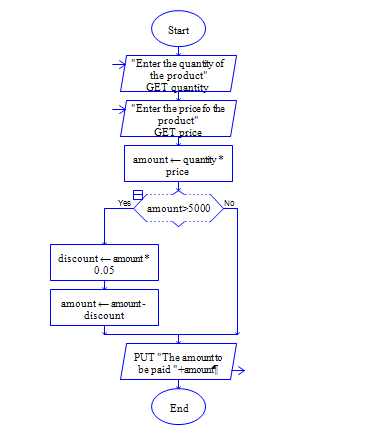# C Program to calculate discount for purchase above 5000

Get price and quantity of a product, calculate bill amount and calculate 10% discount for the bill amount 5000 and above.

Sample Input 1:

10 400

Sample Output 1:

4000

Sample Input 2:

10 500

Sample Output 2:

4500

#### Flow Chart Design#### Program or Solution

``` #include <stdio.h> int main(void) { double quantity,price,amount,discount; printf("Enter Quantity and Price:"); scanf("%lf %lf",&quantity, &price); amount=quantity*price; if(amount>5000) { discount=amount*0.05; amount=amount-discount; } printf("%lf",amount); return 0; } ```

#### Program Explanation

Get two inputs quantity and price from user using scanf statement

Calculate amount by using the expression amount = quantity * price

Check whether amount is greater than 5000 using if statement.

if amount is greater than 5000 then calculate discount and amount using the expressions discount = amount * 0.05 and amount = amount - discount.

print amount using printf statement.